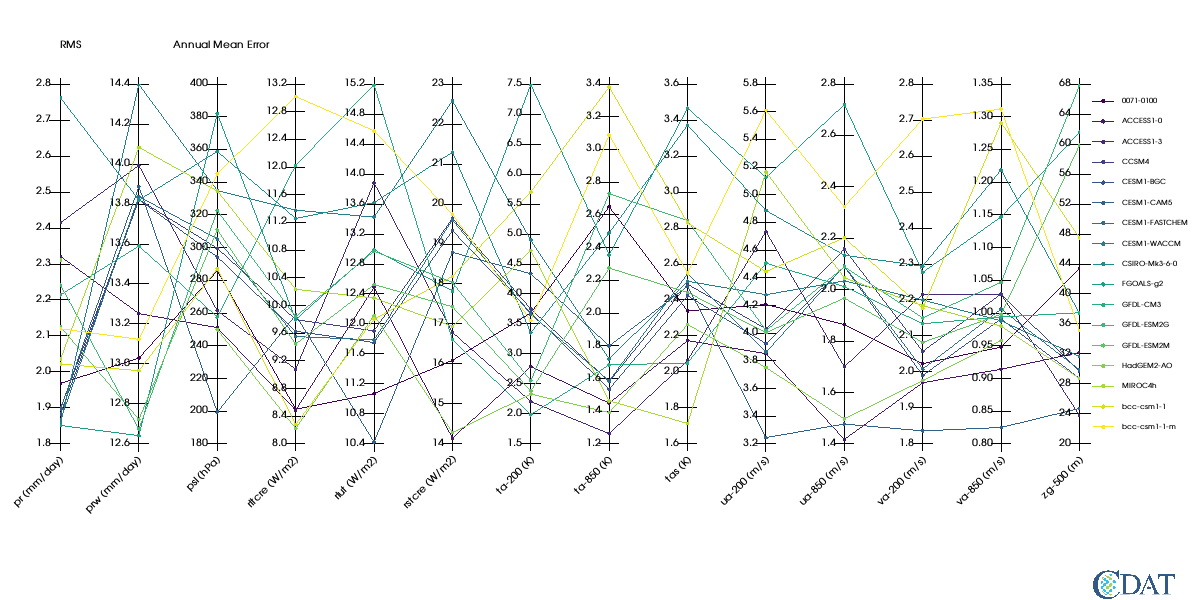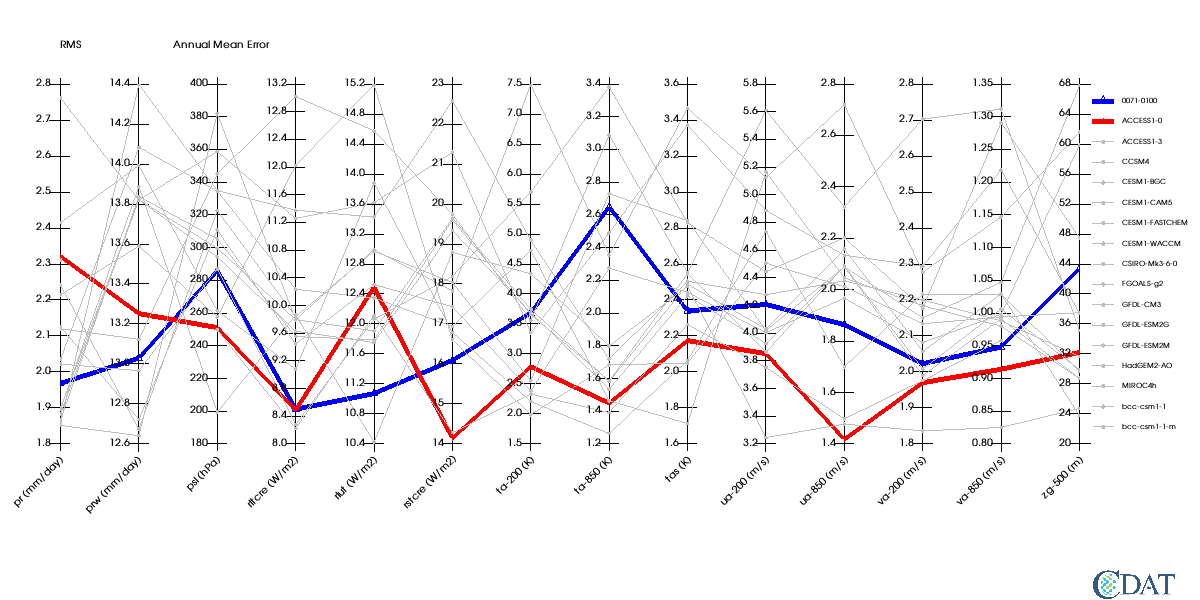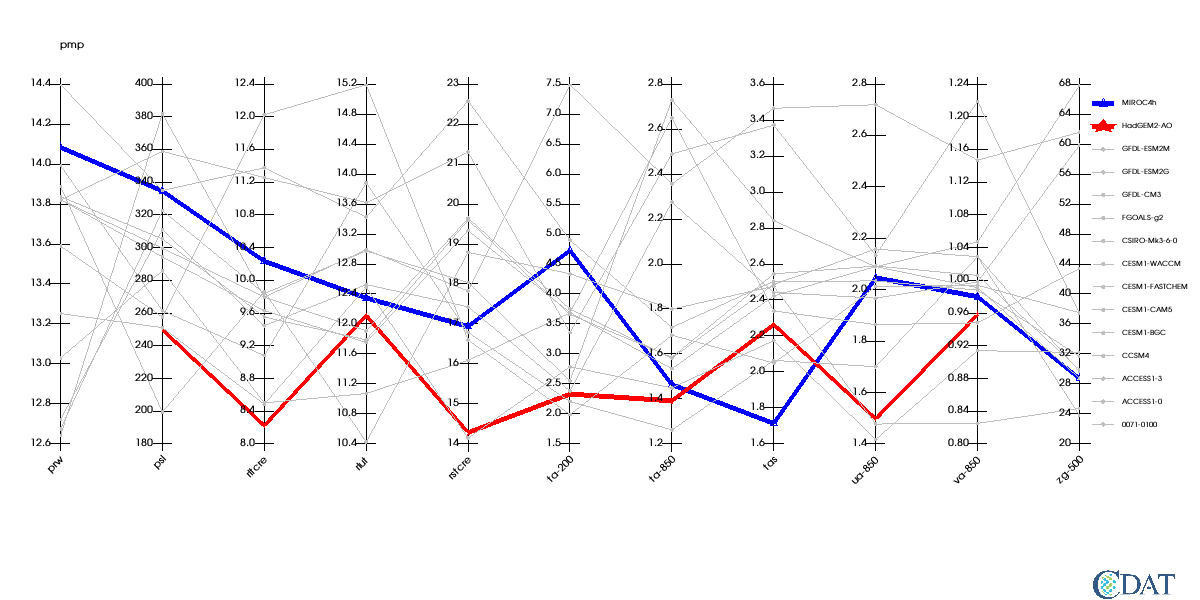import necessary modules

In :
import vcs # For plots
import vcsaddons # module containing pcoords
import cdms2 # for data
import glob # to list files in directories
import pcmdi_metrics # for special json loader class


## Work around to visualize plot in Jupyter Notebook¶

This class allow use to use vcsaddons plots

In :
import tempfile
import base64
class VCSAddonsNotebook(object):
def __init__(self, x):
self.x = x
def _repr_png_(self):
fnm = tempfile.mktemp()+".png"
x.png(fnm)
encoded = base64.b64encode(open(fnm, "rb").read())
return encoded
def __call__(self):
return self



# Sample Data¶

These files are in the test directory of pcmdi_metrics repo at: http://github.com/PCMDI/pcmdi_metrics.git

In :
# Prepare list of json files
import os

# Location on your computer
json_pth = "/git/pcmdi_metrics/tests/graphics"
# Geenrate list ofjson files
json_files = glob.glob(
os.path.join(
json_pth,
"json",
"v2.0",
"*.json"))
json_files += glob.glob(
os.path.join(
json_pth,
"json",
"v1.0",
"*.json"))
# Read them in via pmp special json class
J = pcmdi_metrics.pcmdi.io.JSONs(json_files)

# Retrieve data we need for plot
# Annual mean RMS (XYT dimensions)
# All models and all variables
rms_xyt = J(statistic=["rms_xyt"],season=["ann"],region="global")(squeeze=1)


Let's take a look at the array generated Note the axis are strings of varialbes used and models The order of the axes is the order on the plot

In :
rms_xyt.info()

*** Description of Slab pmp ***
id: pmp
shape: (14, 17)
filename:
missing_value: 1e+20
comments:
grid_name: N/A
grid_type: N/A
time_statistic:
long_name:
units:
tileIndex: None
No grid present.
** Dimension 1 **
id: variable
Length: 14
First:  pr
Last:   zg-500
Other axis attributes:
realtopology: linear
Python id:  0x7f4e29d15a90
** Dimension 2 **
id: model
Length: 17
First:  0071-0100
Last:   bcc-csm1-1-m
Other axis attributes:
realtopology: linear
Python id:  0x7f4e29d154d0
*** End of description for pmp ***

In :
# Ok now let's create a VCS pcoord graphic method

# initialize a canvas
x=vcs.init(geometry=(1200,600),bg=True)
import vcsaddons
gm = vcsaddons.createparallelcoordinates(x=x)


# Preparing the plot¶

## Data¶

'id' is used for variable in plot the JSON class returns var as "pmp", here "RMS" is more appropriate

'title' is used to draw the plot title (location/font controlled by template)

## Template¶

The template section prepares where data will be rendered on plot, and the fonts used

fonts are controlled via textorientation and texttable VCS primary objects

Here we need to angle a bit the xlabels (45 degrees)

We also want to turn off the boxes around the legend and the data area.

In :
# Prepare the graphics
# Set variable name
rms_xyt.id = "RMS"
# Set units of each variables on axis
# This is a trick to have units listed on plot
rms_xyt.getAxis(-2).units = ["mm/day","mm/day","hPa","W/m2","W/m2","W/m2", "K","K","K","m/s","m/s","m/s","m/s","m"]
# Sets title on the variable
rms_xyt.title = "Annual Mean Error"

# Preprare the canvas areas
t = vcs.createtemplate()
# Create a text orientation object for xlabels
to=x.createtextorientation()
to.angle=-45
to.halign="right"
# Tell template to use this orientation for x labels
t.xlabel1.textorientation = to.name

# Define area where plot will be drawn in x direction
t.reset('x',0.05,0.9,t.data.x1,t.data.x2)
ln = vcs.createline()

# Turn off box around legend
ln.color = [[0,0,0,0]]
t.legend.line = ln
# turn off box around data area
t.box1.priority=0

# Define box where legend will be drawn
t.legend.x1 = .91
t.legend.x2 = .99
# use x/y of data drawn for legend height
t.legend.y1 = t.data.y1
t.legend.y2 = t.data.y2

In :
# Plot with default values of graphic method
# Bug vcsaddons need to return a display
# as a result it does not show up in notebook
x.clear()
show = VCSAddonsNotebook(x)
gm.plot(rms_xyt,template=t,bg=True)
show()

Out:# Control various aspects of the graphic method¶

We want the first two model to be 'blue' and 'red' and a bit thicker

All other plots will be 'grey' and 'dashed'

In :
x.clear()
gm.linecolors = ["blue","red","grey"]
gm.linestyles=["solid","solid","dot"]
gm.linewidths=[5.,5.,1.]
gm.markercolors = ["blue","red","grey"]
gm.markertypes=["triangle_up","star","dot"]
gm.markersizes=[1,1,2]
gm.plot(rms_xyt,template=t,bg=True)
show()

Out:In :
# change order and number of models and variables
# We need to creaate a new gm
gm = vcsaddons.createparallelcoordinates(x=x)
axes = rms_xyt.getAxisList()
models = ['MIROC4h', 'HadGEM2-AO', 'GFDL-ESM2M',
'GFDL-ESM2G', 'GFDL-CM3', 'FGOALS-g2', 'CSIRO-Mk3-6-0', 'CESM1-WACCM',
'CESM1-FASTCHEM', 'CESM1-CAM5', 'CESM1-BGC', 'CCSM4', 'ACCESS1-3', 'ACCESS1-0',
'0071-0100'] # invert them
variables = ['prw', 'psl', 'rltcre', 'rlut', 'rstcre', 'ta-200', 'ta-850', 'tas',
'ua-850', 'va-850', 'zg-500']
rms_xyt = J(statistic=["rms_xyt"],season=["ann"],region="global",model=models,variable=variables)(squeeze=1)
x.clear()
gm.linecolors = ["blue","red","grey"]
gm.linestyles=["solid","solid","dot"]
gm.linewidths=[5.,5.,1.]
gm.markercolors = ["blue","red","grey"]
gm.markertypes=["triangle_up","star","dot"]
gm.markersizes=[1,2,2]
gm.plot(rms_xyt,template=t,bg=True)
show()

Out: